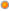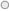## AMAT 42853: Tensor Analysis and General Relativity

User Rating: 4 / 5Course Code    : AMAT 42853

Title                   : Tensor Analysis and General Relativity

Pre-requisites   :  PMAT 21553

Learning Outcomes:

At the end of the course the student should be able to demonstrate knowledge of concepts and theorems in tensor algebra, tensor analysis and the formalism of general relativity, and to solve Einstein’s field equations in simple cases, and to apply the solutions in Astrophysics.

Course Contents:

Covariant and contravariant vectors, Metric tensor, Invariants, Inner products, Differential-forms, Tensor analysis, Covariant differentiation of tensors, Einstein’s field equations, Schwarzchild interior and exterior solutions, Rotating systems, Dragging of inertial frames, Gravitation red shift, Bending of light and gravitational lenses.

Method of Teaching and Learning: A combination of lectures, tutorial discussions and presentations.

Assessment: Based on tutorials, tests, presentations and end of course examination.

1. Adler R., Bazin M. and Schiffer M., Introduction to General Relativity, McGraw Hill, New York. (1975).
2. Misner, C.W.,Thorne, K.S. & Wheeler, J.A., Gravitation, Princeton University Press, 2017.
3. Hawking S.W. & Ellis G.F.R., The Large Scale Structure of Space-time, Cambridge University Press. (1975).
4. James B. Hartle, Gravity: An Introduction to Einstein's General Relativity, Pearson (2013).

## AMAT 42843: Quantum Field Theory

User Rating: 5 / 5Course Code    : AMAT 42843

Title                   : Quantum Field Theory

Pre-requisites   : AMAT 41823

Learning Outcomes:

At the end of this course, the student should be able to demonstrate knowledge of basic properties of relativistic local field theory and the quantization of spin zero and spin half fields.

Course Contents:

Relativistic wave equation, Review of mechanics of a finite system, Quantisation, General Theorems, Quantisation of spin zero fields and spin ½ fields, Momentum and angular momentum operators, Phase factor, Conventions between the spinners, Two - component theory.

Method of Teaching and Learning: A combination of lectures, tutorial discussions and presentations.

Assessment: Based on tutorials, tests, presentations and end of course examination.

1. Schiff, L.I., Quantum Mechanics (4e), McGraw-Hill India. (2014).
2. Prugovecki, E., Quantum Mechanics in Hilbert Space (2e), Courier Corporation, 2013
3. Lee, T. D., Particle Physics and Introduction to Field Theory, Taylor and Francis. (1981).

## AMAT 43976: Research/Study Project

User Rating: 5 / 5Course Code  :           AMAT 43976

Title                :           Research/Study Project

Learning Outcomes:

At the end of this course, the student should be able to demonstrate competence in research/independent-study in an area in Applied Mathematics.

Course Contents:

Undergraduate research project is an inquiry, investigation, or creation produced by a final year honours degree undergraduate that makes a contribution to the discipline and reaches beyond the traditional curriculum. Undergraduate research project is designed to provide students with the opportunity to develop and practice advanced discipline-specific projects in collaboration with senior academics in the department.

Method of Teaching and Learning: A research/study project under the supervision of a senior staff member of the department.

Assessment: Submission of a research/study project report and an oral presentation.

Recommended Reading      : Required reading material will be recommended by the supervisor depending on the relevant project.

## AMAT 41833: Linear programming and Optimization

User Rating: 5 / 5Course Unit Code     : AMAT  41833

Course Title               : Linear programming and Nonlinear Programming

Pre-requisites            : PMAT 21553

Learning outcomes:

Upon successful completion of the course unit the student will be able to:

• develop a linear programming model from problem description,
• use the simplex method for solving linear programming problems,
• use the revised simplex method to solve linear programming problems,
• interpret the dual of a linear programming problem and solve the resulting dual problem using the dual simplex method,
• obtain the solution to the primal problem from the solution of the dual problem
• use methods of linear programming for solving assignment problems and transportation problems
• identify the convex functions
• solve optimization problems in various areas using linear and non-linear programming methods

Course Content:

Optimization, Types of optimization problems,

Linear Programming: Formulate linear programming problem: Extreme Points, Basic Feasible Solutions, Solutions using graphical methods, Simplex method, Revised simplex method, Duality theory, Primal and dual problems, Reduction of linear inequalities, Hungarian method, Big m method.

Non Linear programming: Types of non-linear programing, Convex and concave functions, one variable unconstrained optimization, multivariable unconstrained optimization, Convex programming

Applications of Linear Programming Problems: Transportation problem and Assignment problem

Implement Linear programming problems using Excel

Method of Teaching and Learning: A combination of lectures, tutorial discussions and presentations.

Assessment: Based on tutorials, tests, presentations and end of course examination

Recommended Textbook:

1. David G. Luenberger, Linear and Nonlinear Programming, (4e) Springer; 2016
2. Jiri Matousek, Bernd Gärtner, Understanding and Using Linear Programming. Springer Berlin Heidelberg, 2009
3. S. Hillier and G. Lieberman, Introduction to Operations Research, McGraw-Hill, (10e). 2015

## AMAT 41823: Quantum Mechanics

User Rating: 5 / 5Course Code    : AMAT 41823

Title                   : Quantum Mechanics

Pre-requisites   : AMAT 11513

Learning Outcomes:

At the end of this course, the student should be able to demonstrate knowledge of concepts and principles of quantum mechanics and to apply them to solve simple problems.

Course Contents:

Quantum mechanics in Hilbert space, Axiomatic structure of quantum mechanics, The Shrödinger picture, Heisenberg and interaction picture, Complete set of observables, formalism of wave mechanics and its applications, Completely continuous operators, uncertainty principle, potential well, simple harmonic oscillator, scattering theory of two particles, potential scattering, approximate methods

Method of Teaching and Learning: A combination of lectures, tutorial discussions and presentations.

Assessment: Based on tutorials, tests, presentations and end of course examination.